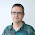## Wednesday, April 16, 2014

### D3 map Styling tutorial IV: Drawing gradient paths

After creating the last D3js example, I was unsatisfied with the color of the path. It changed with the typhoon class at every moment, but it wasn't possible to see the class at every position. When I saw this example by Mike Bostock, I found the solution.

### Understanding the gradient along a stroke example

First, how to adapt the Mike Bostock's Gradient Along Stroke example to a map.
The map is drawn using the example Simple path on a map, from this post. The only change is that the dashed path is changed with the gradient.
You can see the result here.
The differences from drawing a simple path start at the line 100:
var line = d3.svg.line()
.interpolate("cardinal")
.x(function(d) { return projection([d.lon, d.lat]); })
.y(function(d) { return projection([d.lon, d.lat]); });

svg.selectAll("path")
.enter().append("path")
.style("fill", function(d) { return color(d.t); })
.style("stroke", function(d) { return color(d.t); })
.attr("d", function(d) { return lineJoin(d, d, d, d, trackWidth); });

•  The line definition remains the same. From every element it gets, it takes the lat and lon attributes, projecting them, and assigning them to the x and y path properties
• A color function is defined at line 41, which will interpolate the color value from green to red:
var color = d3.interpolateLab("#008000", "#c83a22");
• The data is not the line(track) directly, as in the former example, but passed through the functinos sample and quad.
• The sample function assigns a property t with values between 0 and 1, which is used to get the color at every point.
• Finally, the function lineJoin is used to draw a polygon for the sampled area.
The functions used in the Mike Bostock's example aren't explained, I'll try to do it a little:
• sample takes a line (the data applied to a line function), and iterates with the precision parameter as increment along the string, creating an array with all the calculated points.
• quad takes the points calculated by the sample function and returns an array with the adjacent points (i-1, i, i+1, i+2).
• lineJoin takes the four points generated by quad, and draws the polygon, with the help of lineItersect and perp functions.

### Drawing the typhoon track with the colors according to the typhoon class

The final example draws the typhoon path changing smoothly the color according to the typhoon class.
The animation of the path, and the rotating icon are explained in the third part of the tutorial. In this case, the way to animate the path will change.
For each position of the typhoon, a gradient path is drawn, because the gradient is always between two colors. So the part of the code that changes is:
      //Draw the path, only when i > 0 in otder to have two points
if (i>0){
color0 = color_scale(track[i-1].class);
color1 = color_scale(track[i].class);

var activatedTrack = new Array();

activatedTrack.push(track[i-1]);
activatedTrack.push(track[i]);

var color = d3.interpolateLab(color0, color1);
path_g.selectAll("path"+i)
.enter().append("path")
.style("fill", function(d) { return color(d.t);})
.style("stroke", function(d) { return color(d.t); })
.attr("d", function(d) { return lineJoin(d, d, d, d, trackWidth); });
}

i = i + 1;
if (i==track.length)
clearInterval(animation)

• Inside the animation interval (line 145), the gradient path is create for each position (starting with the second one to have two points)
• The two colors are taken from the point information
• An array with the two points is created, with the name activatedTrack. I tried using more points, but the result is very similar.
• The color interpolation is calculated (line 172)
• The gradient colored path is created (line 173). Note that the name is path+i, to make different paths each iteration, and not to overwrite them. The method is the same as the one used in the first section.
Besides, an invisible path with all the positions is created, so the typhoon icon can be moved as it was in the third part of the tutorial.

1.hi, blogger.
i don't understand what relationship is there between lon/lat and .x/.y
because i want to write circle base on the map .
thanks ^^

2.Hi 小風!

To get a pair of x/y coordinates from a lon/lat, you can use the defined projection function this way:

var coordinates = projection([lon, lat]);

To see how to fill a map with circles, take a look to the last section of this post:

http://www.d3noob.org/2013/03/a-simple-d3js-map-explained.html

I hope this helps!

1.it's useful to me!!
thank you~~^^